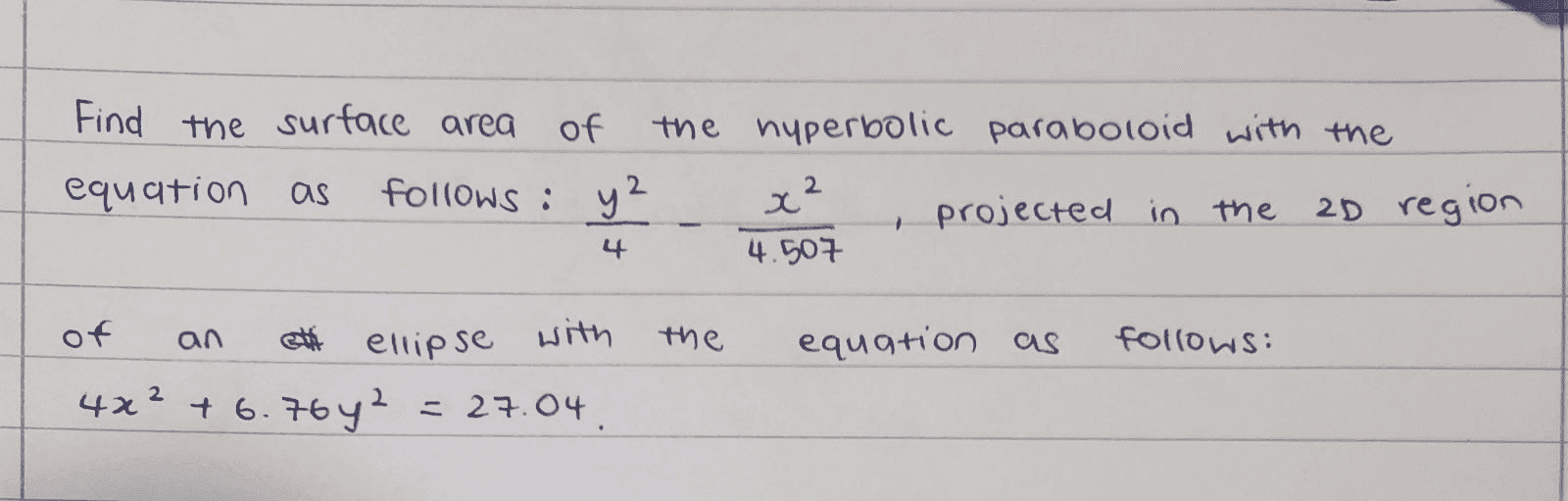# Double integration problem

• MHB
• wonder_koo
In summary, the person is asking for help in solving the surface area of a pringle shape described by the equation z = (y^2)/4 - (x^2)/4.507 within an ellipse. They mention that they have tried various sources but have not been able to find a solution that makes sense. They suggest using parametrization and give the equations for x and y in terms of r and theta, along with the formula for the surface area. They also provide the expression for the magnitude of the cross product between the partial derivatives of r with respect to r and theta.

#### wonder_koo

does anyone know how to solve this/can lead me on a direction to where I will get an answer that actually makes sense lol? I keep getting a negative answer/0. For context, I'm tryna find the surface area of a pringle and all the sources I've visited always estimate the projected 2D region as a circle + rounded off the y and x coefficients to the same number which allows them to simplify to polar coordinates w/ just r in the integration and then solve it BUT this gives only an estimated area of the pringleThe question is:

Find the surface area of the surface $$z = \frac{y^2}{4} - \frac{x^2}{4.507}$$ lying within the ellipse $$\frac{x^2 }{6.76} + \frac{y^2}{4} = 1.$$

You can parametrize the interior of the ellipse $(x/a)^2 + (y/b)^2 = 1$ as $$\begin{split} x &= ar\cos \theta \\ y &= br\sin \theta \end{split}$$ where $0 \leq r \leq 1$ and $0 \leq \theta \leq 2\pi$. Then the surface area of $z = f(r,\theta)$ is given by $$S = \int_0^{2\pi} \int_0^1 \left\| \frac{\partial \mathbf{r}}{\partial r} \times \frac{\partial \mathbf{r}}{\partial \theta}\right\|\,dr\,d\theta$$ where $$\left\| \frac{\partial \mathbf{r}}{\partial r} \times \frac{\partial \mathbf{r}}{\partial \theta}\right\|^2 = b^2\left(\frac{\partial f}{\partial \theta} \sin \theta - \frac{\partial f}{\partial r}r\cos \theta \right)^2 + a^2\left( \frac{\partial f}{\partial r}r\sin \theta + \frac{\partial f}{\partial \theta}\cos\theta \right)^2 + a^2b^2r^2.$$